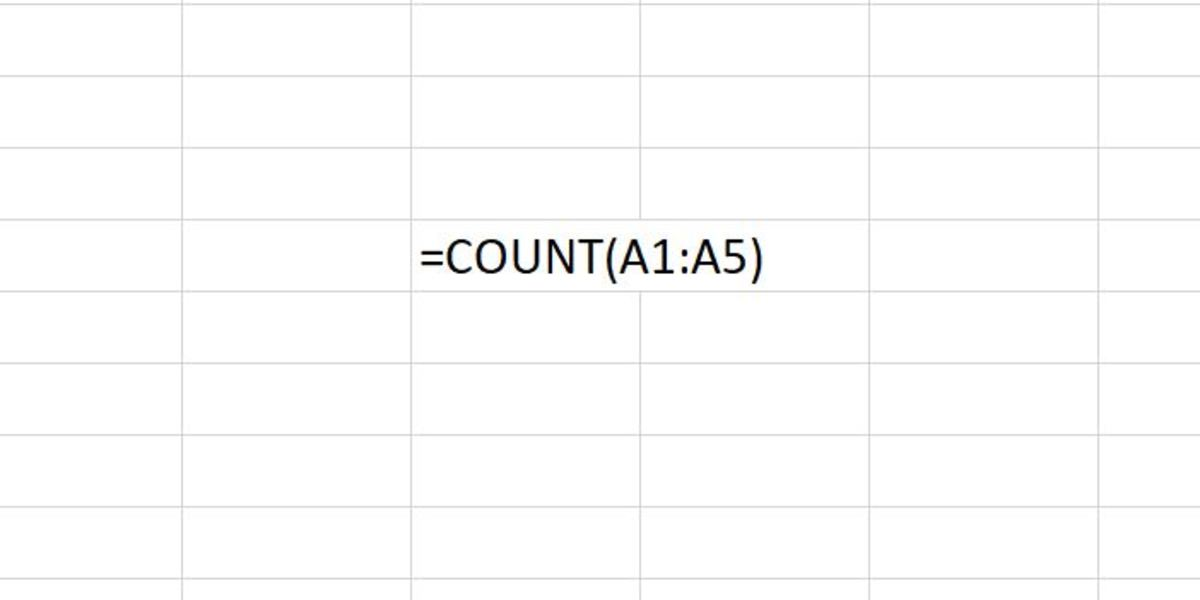# How to Use the COUNT Function in Excel

Joshua earned an MBA from USF and he writes mostly about software and technology.Here the Count function is set to count dates, text representations of numbers, and numbers within the stated range. Created by Joshua Crowder

## The Count Function

The COUNT function counts the number of cells that have numbers in them. More specifically, when cells have dates, text representations of numbers, and numbers in general, cells will be counted.

## The Syntax of the COUNT Function in Excel

The COUNT function follows the formats explained in each section below:

## COUNT the Value for One Cell

=COUNT(A1)

In this situation, the cell will be counted as 1 if the cell meets the numeric criteria to be counted. This might be used when one attribute of a table is binary and dependent if a numeric value is in a cell.

## Count Values in a Range

=COUNT(A1:A5)

In this situation, the cells in the range will be counted and tallied in the cell that the formula is in depending on if each value in the range of cells meets the numeric criteria to be counted. So, if 3 of 5 cells in a range have a numeric value, 3 will appear in that cell the formula was added to.

## Count Values in a Range With Fixed Numbers

=COUNT(A1:A25,20)

In this situation, the cells in the range will be counted depending on if each value in the range of cells meets the numeric criteria to be counted. In addition, a fixed number can be added to the count. More numbers may be added as needed to the count by separating the values with a comma. This may be used when you have fixed numbers that need to be counted with a range that has numeric values that need to be counted. The count from the range may vary depending on if numeric values are added or dropped off of records, but the fixed numbers on the count will remain counted as one per number.

## Count Values in a Named Range

=COUNT(Range1)

Scroll to Continue

This situation is similar to that of counting values in a range. The cells in the named range will be counted and tallied in the cell that the formula is in depending on if values in the named range of cells meet the numeric criteria to be counted. This would be used if the range of cells was named prior to using the count function.

## Entering the COUNT Function Into an Excel Worksheet

When the COUNT function is entered into an Excel worksheet cell it is done so in the form of a formula. This formula can be typed right into a cell by clicking in a cell and first typing "=COUNT(" into the cell. After the open parenthesis, a range, named range or cell needs to be entered depending on the application. This is followed by a close parenthesis. After the formula is created, the enter button can be pressed to create the count in the cell the formula was added to.

The COUNT function can also be inserted into a cell. This is completed by utilizing the formulas tab to insert a function. This method can be started by first selecting a cell. Next, the formulas tab can be clicked, followed by clicking on the "More Functions" button. From the statistical selection, the count can be chosen from the list of functions.

## Select the COUNT Function From the Formulas Tab

A functional argument window will appear and be waiting for input. These fields take the same type of input as the formulas. Ranges, cell references, and numbers can be used to complete up to 255 arguments. Once the OK button is clicked the cells in the arguments will be counted that meet the conditions of the numeric value stated earlier.